## 思路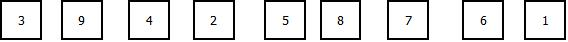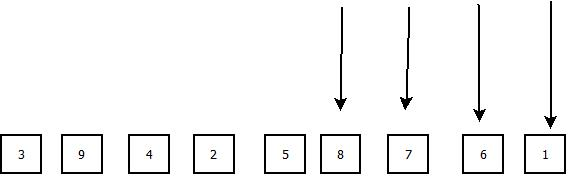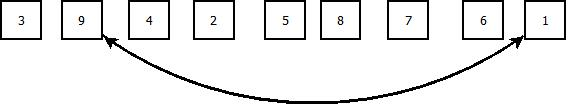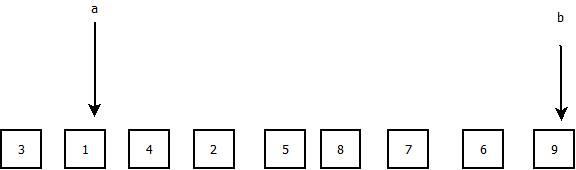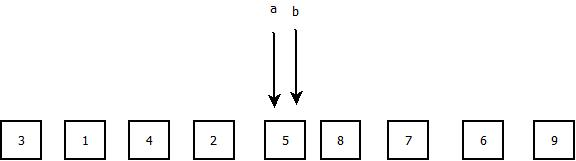a和b现在都指向了我们这个基准的数字5。

## 源码下载

void QuickSortMiddleBase(int array[], int begin, int end) {
if(begin >= end) {
return ;
}
int base_pos = (begin + end) / 2;
int base_value = array[base_pos];

int left_pos = begin, right_pos = end;
int temp_val;

while(left_pos != right_pos) {
while(array[left_pos] < base_value && left_pos <= right_pos) {
left_pos++;
}
while(array[right_pos] > base_value && right_pos >= left_pos) {
right_pos--;
}
if(left_pos <= right_pos) {
temp_val = array[left_pos];
array[left_pos] = array[right_pos];
array[right_pos] = temp_val;
}
}
array[left_pos] = base_value;
PrintArray(array, 9);
QuickSortMiddleBase(array, begin, left_pos - 1);
QuickSortMiddleBase(array, left_pos + 1, end);
}


void QuickSort(int array[], int begin, int end) {
if(begin >= end) {
return ;
}
int base_pos = begin;
int base_value = array[base_pos];

int left_pos = begin, right_pos = end;
int temp_val;
while(left_pos != right_pos) {
while(array[left_pos] < base_value && left_pos < right_pos) {
left_pos++;
}
while(array[right_pos] > base_value && left_pos < right_pos) {
right_pos--;
}
if(left_pos < right_pos) {
temp_val = array[left_pos];
array[left_pos] = array[right_pos];
array[right_pos] = temp_val;
}
}
//array[left_pos] = base_value;
//array[base_pos] = temp_val;
printf("sort result:");
PrintArray(array, 9);
QuickSort(array, begin, left_pos);
QuickSort(array, left_pos + 1, end);

}